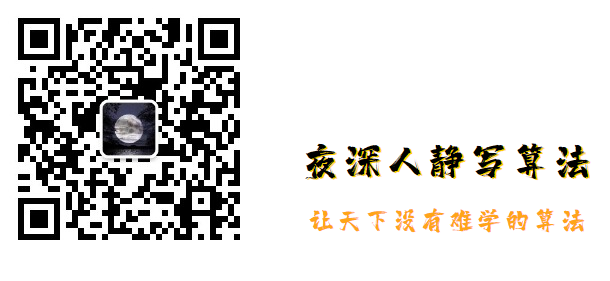• 多重背包 多重背包问题是由0-1背包问题引申而来，在解决多重背包问题之前我们先来了解一下0-1背包问题 0-1背包问题 问题描述：给定n种物品和一背包。物品i的重量是w,其价值为v，背包的容量为C。问:应该如何选择装入...
多重背包
多重背包问题是由0-1背包问题引申而来，在解决多重背包问题之前我们先来了解一下0-1背包问题
0-1背包问题
问题描述：给定n种物品和一背包。物品i的重量是w,其价值为v，背包的容量为C。问:应该如何选择装入背包的物品,使得装入背包中物品的总价值最大?
在选择装人背包的物品时,对每种物品i只有两种选择,即装入背包或不装入背包。不能将物品i装入背包多次，也不能只装入部分的物品i。因此，该问题称为0-1背包问题。
多重背包
①有一个背包，其在n个维度上的容量为 [c1,c2…cn] 。这n个维度可以包括背包载重、背包体积等等属性。
②有m件物品，每件物品i在n个维度上的“重量”为 [w1,w2…wn] ，每件物品i的价值为 v[i] 。
③需要从这m件物品中进行挑选并放入背包，使得物品的总“重量”不超过背包的容量，并让背包中物品的价值最大化。
解决背包问题的常用算法有：
①贪婪算法：计算各物品的性价比（性价比=物品价值/物品“重量”），按照性价比从高到低的顺序选择物品，直至背包中无法放入任何物品为止。
优点：算法简单、求解速度快。
缺点：求得的结果不一定为最优解。
②整数规划：由于数学模型为整数规划，故可用已有的商业求解器解决。
优点：求解速度快、求解结果为最优解。
缺点：需要安装一个求解器。
③动态规划：记录子问题的解，并用子问题的解求出原问题的解。
优点：维度低时求解速度快、求解结果为最优解。
缺点：维度高时求解速度慢、空间复杂度较大（因为要记录子问题的解）。


展开全文• 背包问题的一般情况 —— 多重背包
文章目录一、前言二、多重背包问题1、状态设计2、状态转移方程3、对比 0/1 背包、完全背包问题4、时间复杂度分析三、多重背包问题的优化1、空间复杂度优化2、时间复杂度优化四、多重背包问题的应用1、负权容量2、简单的优化五、多重背包问题相关题集整理
一、前言

如果对 0/1 背包 和 完全背包 已经完全理解，那么今天这一章的内容会显得非常简单，基本上没有太多新的内容，就当温故而知新好了。二、多重背包问题

【例题1】有 $n(n \le 100)$ 种物品和一个容量为 $m(m \le 10000)$ 的背包。第 $i$ 种物品的容量是 $c[i]$，价值是 $w[i]$。现在需要选择一些物品放入背包，每种物品可以选择 $x[i]$ 件，并且总容量不能超过背包容量，求能够达到的物品的最大总价值。

以上就是多重背包问题的完整描述，和 0/1 背包、完全背包的区别就是每种物品的选取有物品自己的值域限制，即文中红色字体标注的内容；

1、状态设计

第一步：设计状态；
状态 $(i, j)$ 表示前 $i$ 种物品恰好放入容量为 $j$ 的背包 $(i \in [0, n], j \in [0, m])$；
令 $dp[i][j]$ 表示状态 $(i, j)$ 下该背包得到的最大价值，即前 $i$ 种物品（每种物品可以选择 $x[i]$ 件）恰好放入容量为 $j$ 的背包所得到的最大总价值；

2、状态转移方程

第二步：列出状态转移方程；$dp[i][j] = max(dp[i-1][j - c[i]*k] + w[i]*k) \\ (0 \le k \le x[i])$
因为每种物品有 $x[i]$ 种可放置，将它归类为以下两种情况：
1）不放：如果 “第 $i$ 种物品不放入容量为 $j$ 的背包”，那么问题转化成求 “前 $i-1$ 种物品放入容量为 $j$ 的背包” 的问题；由于不放，所以最大价值就等于 “前 $i-1$ 种物品放入容量为 $j$ 的背包” 的最大价值，对应状态转移方程中 $k = 0$ 的情况， 即 $dp[i-1][j]$；
2）放 k 个：如果 “第 $i$ 种物品放入容量为 $j$ 的背包”，那么问题转化成求 “前 $i-1$ 种物品放入容量为 $j-c[i]*k$ 的背包” 的问题；那么此时最大价值就等于 “前 $i-1$ 种物品放入容量为 $j-c[i]*k$ 的背包” 的最大价值 加上放入 $k$ 个第 $i$ 种物品的价值，即 $dp[i-1][j - c[i]*k] + w[i]*k$；
枚举所有满足条件的 $k$ 就是我们所求的 “前 $i$ 种物品恰好放入容量为 $j$ 的背包” 的最大价值了。

3、对比 0/1 背包、完全背包问题

多重背包问题是背包问题的一般情况，每种物品有自己的值域限制。如果从状态转移方程出发，我们可以把三种背包问题进行归纳统一，得到一个统一的状态转移方程如下：$dp[i][j] = max(dp[i-1][j - c[i]*k] + w[i]*k)$
对于 0/1 背包问题，$k$ 的取值为 $0,1$；
对于完全背包问题，$k$ 的取值为 $0, 1, 2, 3, ..., \lfloor \frac j {c[i]} \rfloor$；
对于多重背包问题，$k$ 的取值为 $0, 1, 2, 3, ..., x[i]$；

4、时间复杂度分析

对于 $n$ 种物品放入一个容量为 $m$ 的背包，状态数为 $O(nm)$，每次状态转移的消耗为 $O(x[i])$，所以整个状态转移的过程时间复杂度是大于 $O(nm)$ 的，那么实际是多少呢？
考虑最坏情况下，即 $x[i] = m$ 时，那么要计算的 $dp[i][j]$ 的转移数为 $j$，总的状态转移次数就是 $\frac {m(m + 1)} {2}$，所以整个算法的时间复杂度是 $O(nm^2)$ 的，也就是说状态转移均摊时间复杂度是 $O(m)$ 的。
接下来一节会对多重背包问题的空间复杂度和时间复杂度进行优化。

三、多重背包问题的优化
1、空间复杂度优化

一个容易想到的优化是：我们可以将每种物品拆成 $x[i]$ 个，这样变成了 $\sum_{i=1}^n x[i]$ 个物品的 0/1 背包问题，我们知道 0/1 背包问题优化完以后，空间复杂度只和容量有关，即 $O(m)$。
所以多重背包问题的空间复杂度至少是可以优化到 $O(m)$ 的。

2、时间复杂度优化

然而， 如果这样拆分，时间复杂度还是没有变化，但是给我们提供了一个思路，就是每种物品是可以拆分的。
假设有 $x[i]$ 个物品，我们可以按照 2 的幂进行拆分，把它拆分成：$1, 2, 4, ..., 2^{k-1}, x[i] - 2^{k} + 1$
其中 $k$ 是最大的满足 $x[i] - 2^{k} + 1 \ge 0$ 的非负整数。
这样，1 到 $x[i]$ 之间的所有整数都能通过以上 $k+1$ 个数字组合出来，所以只要拆成以上 $k+1$ 个物品，所有取 $k (0 \le k \le x[i])$ 个物品的情况都能被考虑进来。
举例说明，当 $x[i] = 14$ 时，可以拆分成 1,2,4,7 个物品，那么当我们要取 13 个这类物品的时候，相当于选择 2、4、7，容量分别为 $c[i]*2, c[i]*4, c[i]* 7$, 价值分别为 $w[i]*2, w[i]*4, w[i]* 7$。
通过这种拆分方式，$x[i]$ 最多被拆分成 $log_2 {x[i]}$ 个物品，然后再用 0/1 背包求解，得到了一个时间复杂度为 $O(m\sum_{i=1}^{n}log_2{x[i]})$ 的算法。

四、多重背包问题的应用
1、负权容量

【例题2】你手上 $n(n \le 100)$ 种不同的货币，第 $i$ 种货币的价值为 $v[i] (v[i] \le 120)$，个数为 $c[i] (0 \le c[i] \le 10000)$ 个， 现在你去商场里面买一件价值为 $m(m \le 10000)$ 的商品，希望 花费的货币 和 找回的货币 总和最少(假设商场的货币无限多)。

因为涉及到找回，所以引入一个负容量的概念。
对每种货币增加一种对应的负价值货币，数量无限个（可以定一个比较大的值，比如 1000000 ），然后对物品进行二进制拆分。
$dp[i][j]$ 表示前 $i$ 种货币组合出 $j$ 的价值的最小货币个数，则有状态转移方程：
$dp[i][j] = min( dp[i-1][j], dp[i-1][ j - v[i] ] + cnt[i] )$
这是个 0/1 背包的状态转移方程，我们对它进行降维，得到：
$dp[j] = min( dp[j], dp[ j - v[i] ] + cnt[i] )$
我们发现，这里的 $v[i]$ 有可能是负数，所以状态转移的顺序很重要，分情况进行求解：
1）$v[i] > 0$ 时，逆序求解；
2）$v[i] < 0$ 时，顺序求解；
现在来讨论可能达到的最大容量是多少，考虑这么一种情况，假设有两种货币，价值分别为 120 和 119，并且你手上 120 的货币有 10000 个，119 的货币为0个，现在需要买一个价值为 10000 的物品，那么我们可以列出一个方程：
$120x - 119y = 10000$
其中 $x$ 表示付出的价值为 120 的货币数，$y$ 表示找回的价值为 119 的货币数，这个方程是一个线性同余方程，$gcd(120,119)=1$，所以一定是有解的。状态转移过程中能够达到的最大容量应该是 $120x$，并且要保证 $120x - 10000$ 是 119 的倍数，回归一般情况，这里的 119 也可能是 117, 113 等等。所以取最大容量为 $m + 120*120$。

2、简单的优化

【例题3】有 $n(n \le 100)$ 种物品和一个容量为 $m(m \le 100000)$ 的背包。第 $i$ 种物品的容量是 $c[i](c[i] \le 100)$，价值是 $w[i]$。现在需要选择一些物品放入背包，每种物品可以选择 $x[i](x[i] \le 10)$ 件，并且总容量不能超过背包容量，求能够达到的物品的最大总价值。

这个问题有个特点是单个物品容量和背包最大容量相差较大，比如 10 个容量为 100 的物品最多只能填满一个容量为 1000 的背包，所以我们可以对拆分后的物品的容量从小到大排序，然后在进行 0/1 背包时，对最大容量进行累加，令枚举到当前 $i$ 个物品的时候容量总和为 $s[i]$ ，那么大于这个值的状态不用求，实际上我们只需要求最多为 $min(s[i], m)$ 的状态。

关于 多重背包 的内容到这里就结束了。
如果还有不懂的问题，可以 想方设法 找到作者的微信进行在线咨询。

本文所有示例代码均可在以下 github 上找到：github.com/WhereIsHeroFrom/Code_Templates五、多重背包问题相关题集整理

题目链接
难度
解析

HDU 1059 Dividing
★☆☆☆☆
组合问题

PKU 1276 Cash Machine
★☆☆☆☆
组合问题

★☆☆☆☆
组合问题

HDU 1171 Big Event in HDU
★☆☆☆☆
组合问题

HDU 2021 发工资咯：）
★☆☆☆☆
组合问题

HDU 2191 珍惜现在，感恩生活
★☆☆☆☆
【例题1】最值问题

洛谷P1776 宝物筛选
★★☆☆☆
二进制拆分

洛谷P1833 樱花
★★☆☆☆
二进制拆分

HDU 2844 Coins
★★☆☆☆
组合问题

HDU 3732 Ahui Writes Word
★★☆☆☆
二进制拆分

PKU 1787 Charlie’s Change
★★☆☆☆
路径回溯

HDU 3348 coins
★★☆☆☆
特殊多重背包的搜索求解

PKU 3260 The Fewest Coins
★★★☆☆
【例题2】负权多重背包

HDU 3591 The trouble of Xiaoqian
★★★☆☆
【例题2】负权多重背包

HDU 5527 Too Rich
★★★☆☆
特殊多重背包的搜索求解

PKU 1742 Coins
★★★☆☆
楼教主 男人八题 之一


展开全文算法 动态规划 背包问题 数据结构...